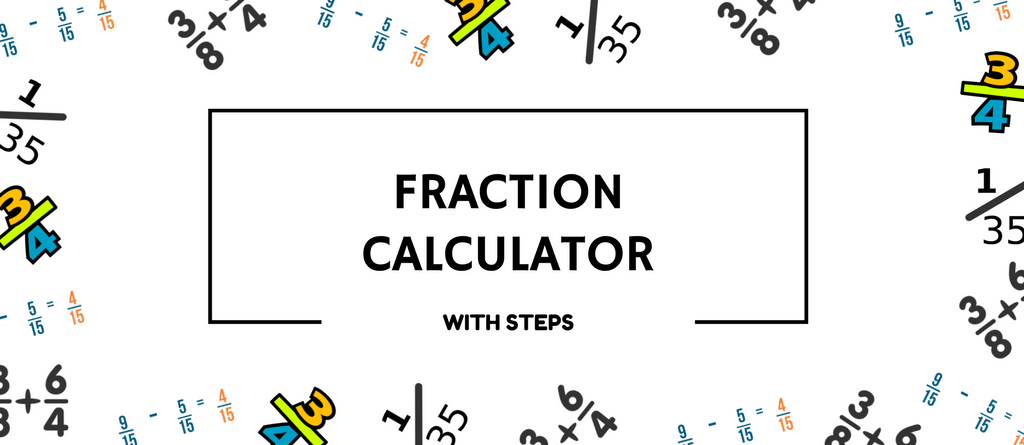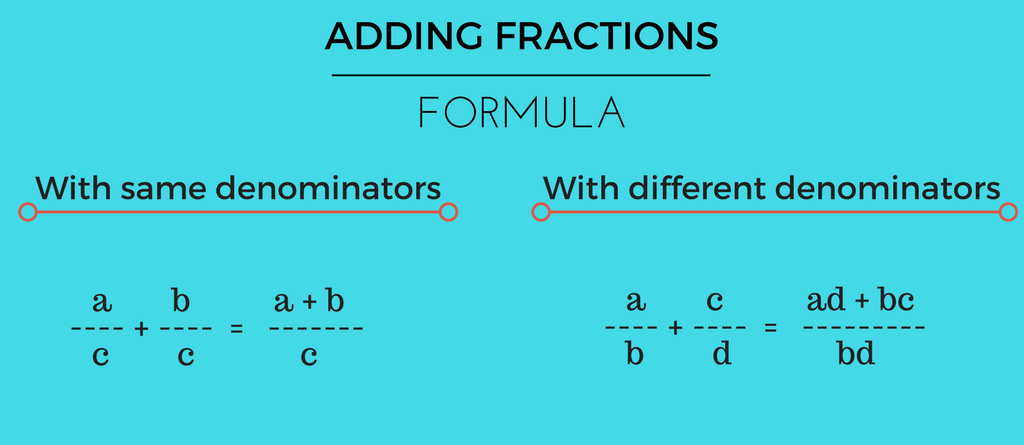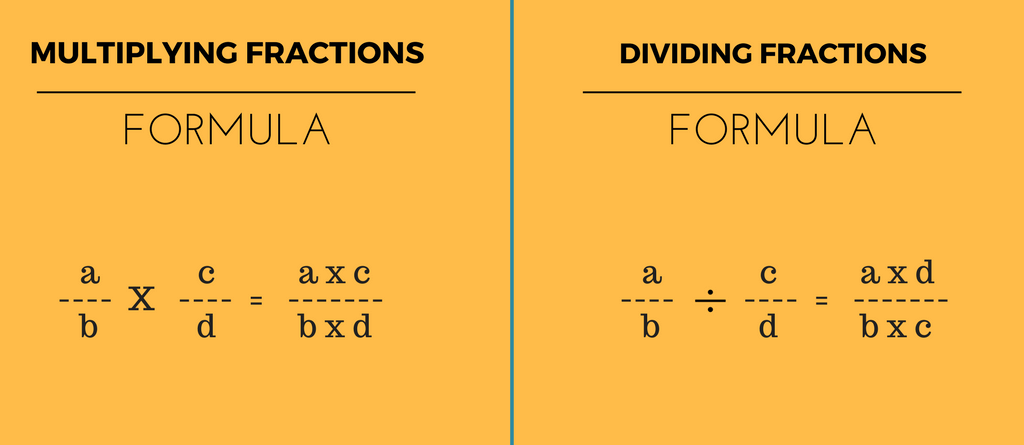### Online Fraction Calculator

Simple! Put the numbers in the spaces below and choose the mathematical symbol from the drop-down menu to get the answer. See the step by step solution for your fraction sum by clicking on ‘hint’.

Trouble understanding fractions? See the formula and step – by – step explanation of each operation in fraction. Let’s start with adding fractions. To see other operations, click the links below:

Subtracting fractions

Multiplying fractions

Dividing fractions.

Know the step by step solution of adding fractions.

### Formula#### Step By Step Solution

Let’s take an example –

 1 + 3 5 5

Solution:

##### Step 1: Equating the denominators
 1 x 5 = 5 5 x 5 25
 3 x 5 = 15 5 x 5 25
##### Step 2: Adding numerators:

5 + 15 = 20

Resulting Fraction:

 20 25
##### Step 3: Simplify

Factors of 20 is – 1, 2, 4, 5, 10, 20

Factors of 25 is – 1, 5, 25

Common Factors of 20 and 25 are –  1, 5

Division by Greatest Common Factor

 20 5
 25 5

Reduced Fraction is

 4 5

Why just stop at a simple calculation? Understand the concept with step by step solution for adding different types of fractions. It is not too hard. Learn how to add fractions in simple steps.

## Subtracting Fractions

Know subtraction fractions in 3 simple steps. We have explained the steps for your understanding. So, subtract your fraction problems away with above free online fraction calculator and get your answer with their step by step solutions for subtracting fractions.

### Formula#### Step By Step Solution

Let’s take an example – 3/4 – 1/4

Solution:

 3 – 1 4 4

Solution:

##### Step 1: Equating the denominators
 3 x 4 = 12 4 x 4 16
 1 x 4 = 4 4 x 4 16
##### Step 2: Adding numerators:

12 – 4 = 8

Resulting Fraction:

 8 16
##### Step 3: Simplify

Factors of 8 is – 1, 2, 4, 8

Factors of 16 is – 1, 2, 4, 8, 16

Common Factors of 8 and 16 are –  1, 2, 4, 8

Division by Greatest Common Factor

 8 8
 16 8

Reduced Fraction is

 1 2

Read ‘How to subtract fractions in 3 simple steps’ to learn more about subtracting different types of fractions. Understand the concept and apply to subtract fractions without fractions calculator.

## Multiplying Fractions

Multiplying fractions is as easy as adding and subtracting fractions. Just put the numbers in the spaces given below and multiply the fractions.

### Formula#### Step By Step Solution

Let’s take an example –

 1 + 3 5 2

Solution:

##### Step 1:Multiply the numerator of both fraction as new numerator and multiply the denominator of both fraction as new denominator
 1 x 3 = 3 5 x 2 10
##### Step 2:Simplify:
Factors of 3 is: 1,  3.
Factors of 10 is: 1,  2,  5,  10
Common Factors of 3 and 10 are: 1
Division by Greatest Common Factors
 3 1
 10 1
##### Resulting Fraction is
 3 10

Don’t just stop here. Understand the concept of multiplying fractions in simple steps with examples. It won’t take more than 2 minutes!

## Dividing Fractions

Dividing fractions has been a monstrous task for kids with too many steps and technicalities involved in it.
Well, now you don’t have to worry anymore because the above Fraction Calculator will not only solve your fraction problems but also give you the simple steps involved in dividing fractions.

#### Step By Step Solution

Let’s take an example – 2/4 divided by 3/5

Solution:

 2 ÷ 3 4 5

Solution:

##### Step 1: Multiply the numerator of one fraction with the denominator of the other
 2 x 5 = 10 4 x 3 12
##### Step 2: Find common factors:

Factors of 10 is – 1, 2, 4, 5, 10,

Factors of 12 is – 1, 2, 3, 4, 6, 12

Common Factors of 10 and 12 are –  1, 2

##### Step 3: Division by common factor
 10 2
 12 2

Reduced Fraction is

 5 6

Easy right? Well, even understanding ‘how to divide fractions‘ is also not that hard. Know the step by step solution of dividing fractions with examples.

But wait, is it everything you need? How about some fun math games for fractions and math worksheets for better practice and experience.  Download free math worksheets from our vast collection of printable. Also, check out fraction games like Monkey Fractions and Froggy Fractions (also available on Amazon).

Other FREE online calculator available –

This calculator with fractions as it’s main mathematical skill, can be used to check your answer to complex fraction sums. So, here’s hoping that this fraction calculator helps you in coming up with quick solutions.